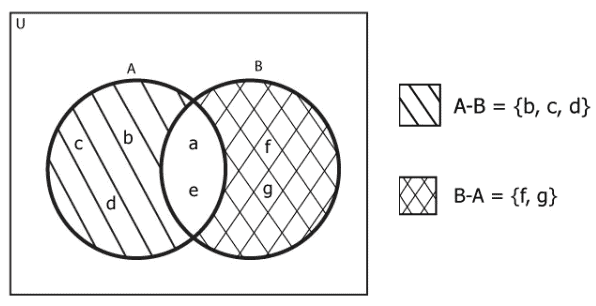# Python Set Difference: The Complete Guide

Python set supports four great operations:

1. Intersection: Elements two sets have in common.
2. Union: All the elements from both sets.
3. Difference: Elements are present on one set but not on the other.
4. Symmetric Difference: Elements from both sets that are not present on the other.

Consider the following image for better understanding.Here, A contains {a,b,c,d,e} and B contains {a,e,f,g} . So A-B={b,c,d} and B-A={f,g} .

## Python Set Difference

Python set difference() is a built-in function that helps us find the difference between two sets. It works like a math intersection (A∩B). This means that if A and B are two sets, then their difference will be:

A-B = A-(A∩B)

B-A = B-(A∩B)

The difference() method returns the set difference of two sets. If A and B are two sets. The set difference between A and B is a set of elements that exist only in set A but not in B.

### Syntax

`First_Set.difference(Second_Set)`

The above syntax will help us to find the difference between First_Set-Second_Set.

So, If we want to find A-B, the syntax will be the following.

`A.difference(B)`

### Return Value

The difference() function returns the difference of two given set without changing the original sets. This means it returns a new set.

### Programming Example

See the following code example.

```# app.py

# Declaring two sets
A = {'a', 'b', 'c', 'd', 'e'}
B = {'a', 'e', 'f', 'g'}

# Now we will apply difference() to find

# A-B
print("Difference between A-B is: ", A.difference(B))
# B-A
print("Difference between B-A is: ", B.difference(A))
```

#### Output

```Difference between A-B is:  {'c', 'b', 'd'}
Difference between B-A is:  {'g', 'f'}
```

Here in this example, we have declared two sets, A and B, and then we have used the difference() method to find the difference between the two sets.

## Using the minus (-) operator

See the following code.

```# app.py

# Declaring two sets
A = {'a', 'b', 'c', 'd', 'e'}
B = {'a', 'e', 'f', 'g'}

# Now we will apply the minus operator to find

# A-B
print("Difference between A-B is: ", A-B)
# B-A
print("Difference between B-A is: ", B-A)
```

#### Output

```Difference between A-B is:  {'d', 'c', 'b'}
Difference between B-A is:  {'g', 'f'}
```

Here in this example, we have declared two sets, A and B, and then we have used the minus operator (-) to find the difference between the two sets.

The original sets are not changed; it remains unchanged.

The difference between the two sets in Python equals the difference between the number of elements in the two sets.

That’s it for this tutorial.